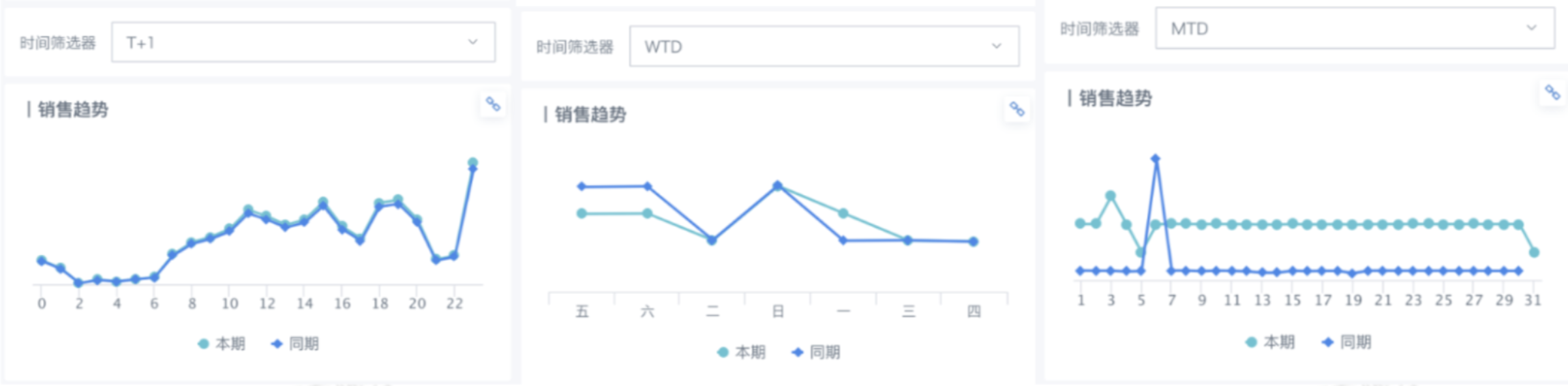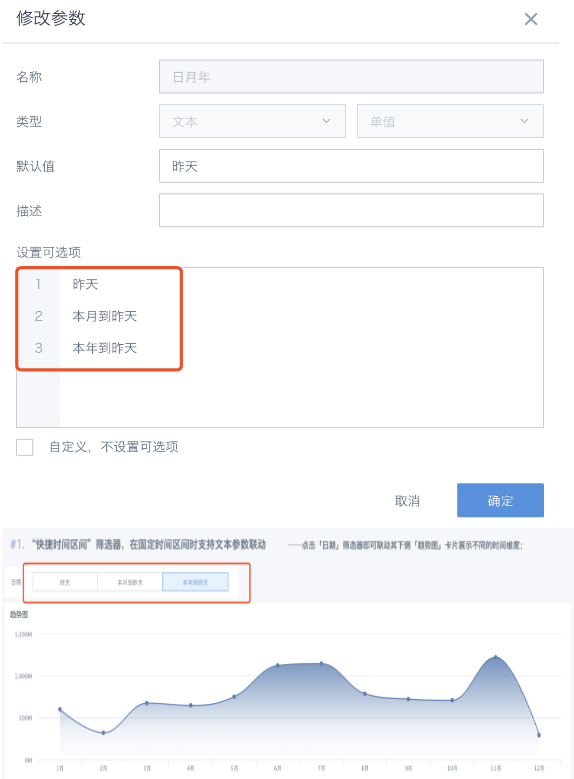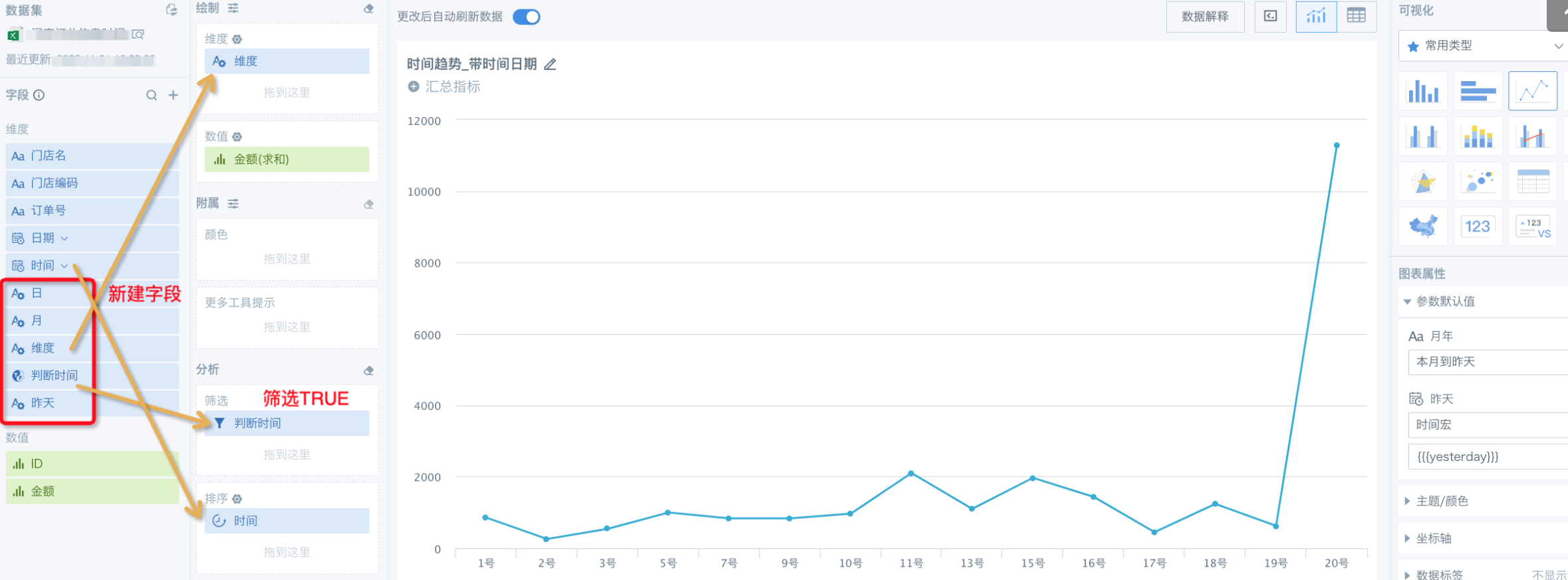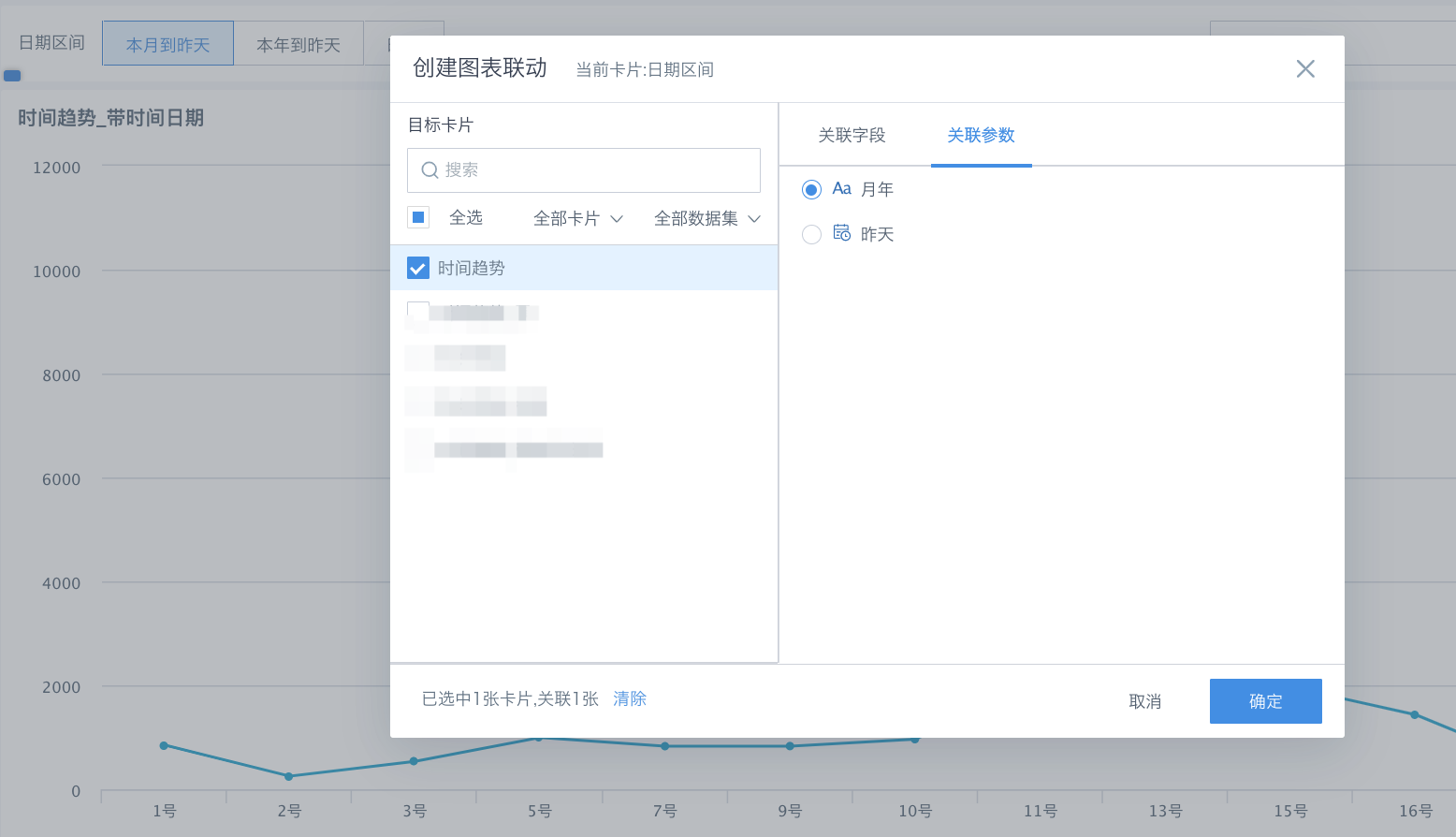# 在固定时间区间时支持文本参数联动

## 需求：“快捷时间区间”筛选器，在固定时间区间时支持文本参数联动## 实现方式：

【建立全局参数】```判断时间：
case when [DYNAMIC_PARAMS.月年]="本月到昨天"
then
(case when [时间] between DATE_TRUNC('month',[DYNAMIC_PARAMS.昨天]) and CURRENT_DATE() then true else false end)
when [DYNAMIC_PARAMS.月年]="本年到昨天" then
(case when [时间] between DATE_TRUNC('year',[DYNAMIC_PARAMS.昨天]) and CURRENT_DATE() then true else false end)
when [DYNAMIC_PARAMS.月年]="昨天" then
(case when cast([时间] as date)=CURRENT_DATE()-1 and hour([时间])<=hour(now()) then true else false end )
else false end```
```日：
case when [日期] between DATE_TRUNC('month',[DYNAMIC_PARAMS.昨天]) and [DYNAMIC_PARAMS.昨天] then CONCAT(right([日期],2),'号') else null end```
```月：
case when YEAR([时间])=YEAR(CURRENT_DATE()) and [时间]<=CURRENT_DATE() then CONCAT(MONTH([时间]),'月') else null end```
```昨天：
case when cast([时间] as date)=CURRENT_DATE()-1 and hour([时间])<=hour(now())
then CONCAT(hour([时间]),'点') else null end```1 人点赞过